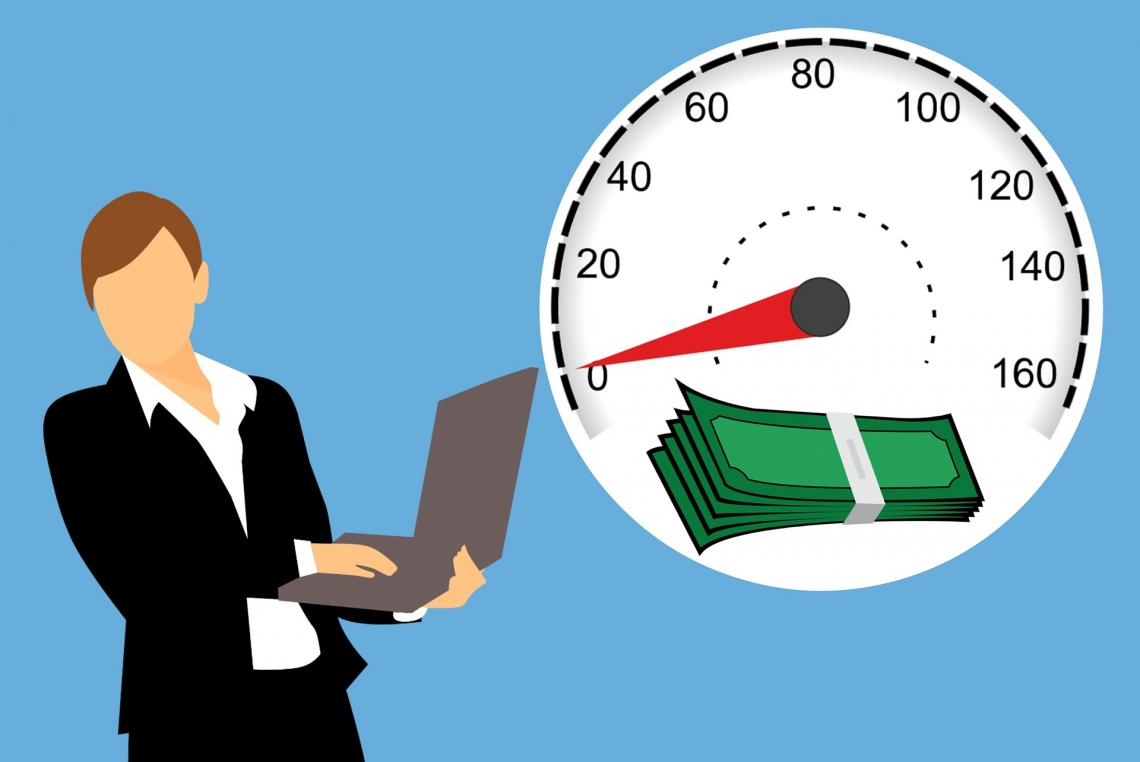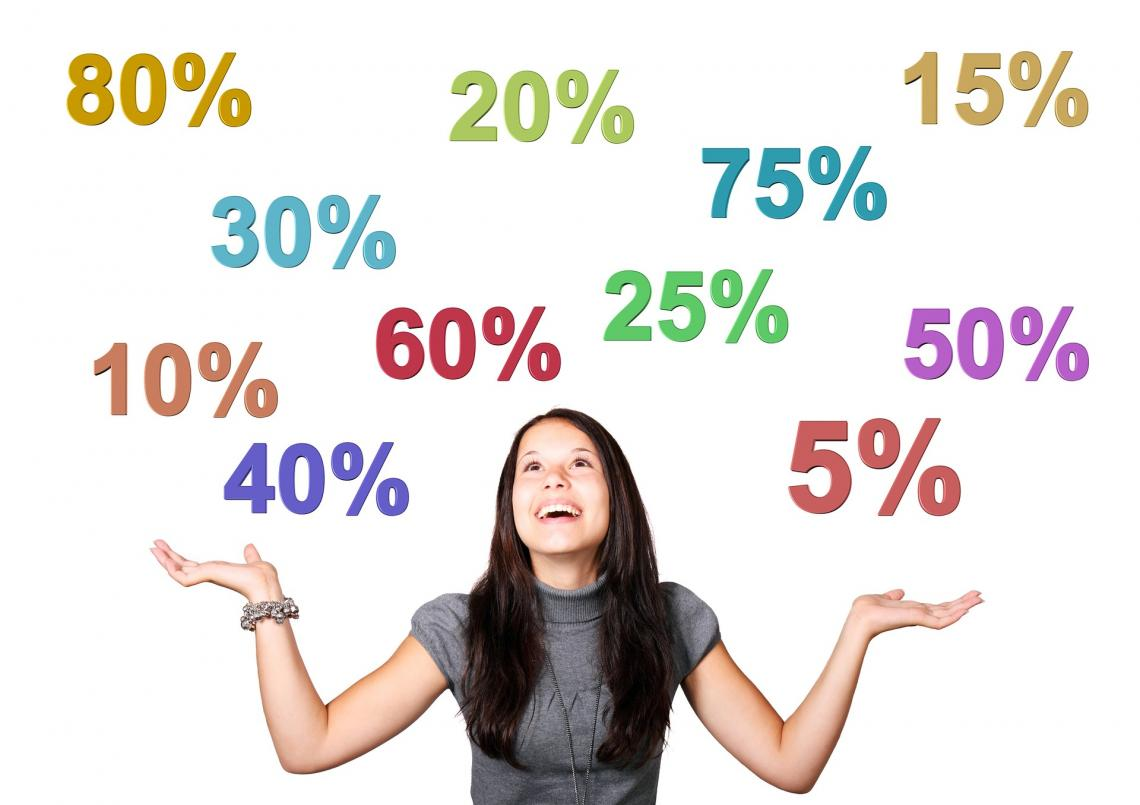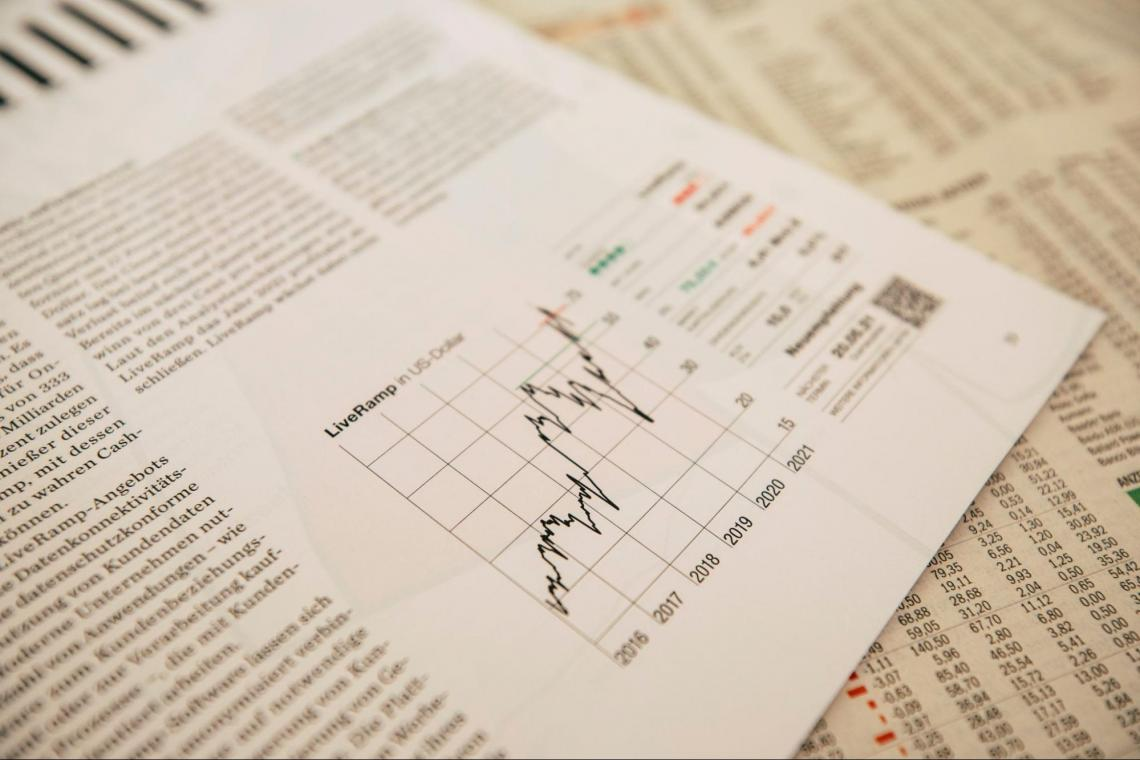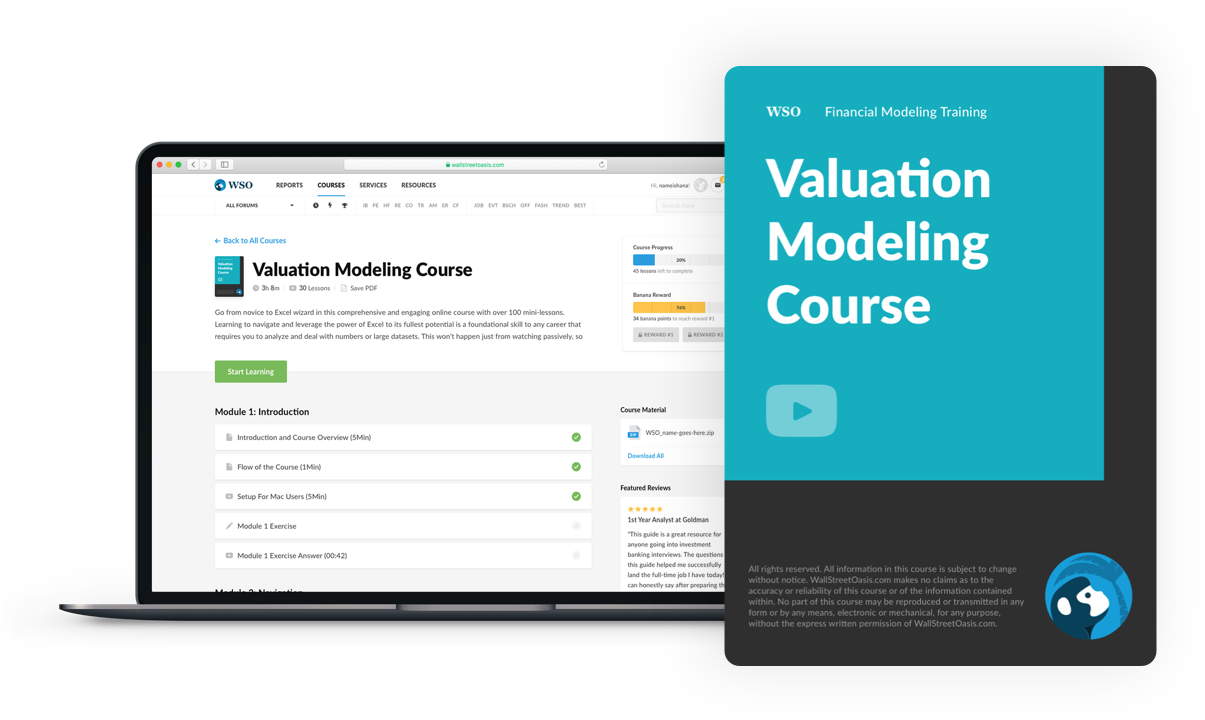# Notional Value

Refers to the total value of a derivatives trade and is the product of total units traded and the spot price.

Any financial instrument's notional value(NV), which is determined by multiplying the total number of units in the contract by the current market spot price for the relevant units, represents the total value of the derivative contract it holds.It is the total underlying amount of a derivatives trade-in market parlance.

Because of a concept known as leverage, the notional value of derivative contracts is much higher than the market value. Leverage allows a small amount of money to control a much larger amount of money theoretically.

As a result, it aids in distinguishing the total value of a trade from the cost (or market value) of the trade.

There is a distinct difference: the market value is the price at which the position can be bought or sold in the market, whereas the notional value represents the position's total value.

Based on the spot price, a financial instrument's NV represents the underlying securities' total value. Similar terms are employed in a variety of derivative contracts, including interest-rate swaps, currency swaps, stock options, and others.The stock trading term "notional value," also known as "notional amount," is frequently used in the context of valuing the underlying assets during a derivatives trade.

It could be the total value of a given position, the amount of value controlled by a position, or a contractually agreed-upon, predetermined amount.

When referring to derivative contracts in the futures, options, and currency markets, the term "notional value" is frequently used.

Let's examine a notional amount or value in the context of the stock market now that its definition has been provided.

The total amount of derivatives trading is simply referred to as notional value. Because of a trading concept known as leverage, the notional amount or value of a derivatives contract is typically much higher than its market value.

## Notional Value Vs. Market Value

A security's worth can be expressed in terms of its market value or notional value.By way of debt obligations or derivatives contracts, for example, a security may theoretically control a certain amount of total value, referred to as notional value.

On the other hand, market value is the price at which a security can currently be purchased or sold through a broker or an exchange.

Market value, which is calculated by dividing the number of outstanding shares by the current share price, is also used to describe the market capitalization of a publicly-traded company.

Theoretically, a security's NV represents how much it controls. It is typically greater than the market value.

Equity options and currency swaps both use NV. The value on which interest rate payments will be exchanged is determined by using this formula. Additionally helpful in determining portfolio risk is the NV.

The price at which securities can be bought and sold is referred to as market value. It refers to a security's actual market value at the time the security is purchased. Market value is also used to calculate a company's market capitalization.

The market price of a share, or the current share price, can be multiplied by the number of outstanding shares to determine this value.

## Relevance

The total value of a security's underlying asset at market value is known as the NV. It makes a distinction between the investment amount and the overall sum of the payment.By multiplying the units in a single contract by the spot price, the NV is determined.

Consider the following comparison of a bond and an interest rate swap:

• The purchaser of a bond pays the principal amount at issue (start), then receives coupons (calculated off this principal) over the life of the agreement, and finally gets the principal back at maturity (end).

• No principal exchanges hands during the start or end of a swap, so the term "notional principal amount," also known as "notional," was created to describe the amount that acts as if it were the bond's principal. Interest payments are computed based on this notional amount during this time.

• The notional principal amount is essentially the number of assets or bonds that a person owns.

## Important Terms

When describing the value of the underlying asset in a derivatives trade, the term "notional value" is frequently employed. A position's overall value, the quantity of value it controls, or a contract's predetermined sum are all examples of the position value. In the options, futures, and currency markets, such terminology is being used to describe derivative contracts.

1. Equity Options

A holder of an equity option has the right, but not the obligation, to buy or sell the underlying security at the strike price in the future.

The total value of the options that a shareholder owns is indicated by the option's nominal value.

2. Interest Rate Swaps

A contract in which the parties agree to exchange future interest payments is known as an interest rate swap.

A notional principal amount that is decided upon in advance is used to calculate interest. Interest is calculated by multiplying the applicable interest rate by the notional principal amount. Consequently, interest calculations start with this value.The notional principal amount, which can be 8000 US dollars, 2.7 million pounds sterling, or any other number and currency combination, is the specified sum on which the exchanged interest payments are based in an interest rate swap.

To determine the height and currency of each counter-payment, the party's rates for each period are multiplied by the notional principal amount.

An "interest-only class" that is not entitled to any principal is given a "notional principal amount" as a basis for calculating the amount of interest due.

3. Currency Swaps

A contract in which the parties agree to swap the principal sum and any future interest payments made in different currencies is known as a currency swap.

It aids in the calculation of interest payments on the predetermined notional principal in currency swap contracts, just as it does in interest rate swaps.

4. Total Return Swaps

In total return swaps, a party is required to pay a floating or fixed rate multiplied by the NV amount plus the NV decline. By paying the increase in NV, one party exchanges this for payments from another.

## Examples

The following examples can help you better understand this concept, which can be tricky at first.

Example #1

When referring to an option's notional value, we mean the value that it controls. For instance, WSO is currently trading at \$20, and a specific WSO call option costs \$1.50.

There are 100 underlying shares under one equity option.

1. A trader pays \$1.50 x 100 = \$150 for the option.
2. The option's NV is \$20 x 100 = \$2,000.
3. Instead of paying \$2,000 for 100 shares of stock outright, the trader could potentially control them for \$150 by purchasing a stock option contract.
4. Instead of the price of the transaction, the NV of an equity options contract is the value of the shares that are controlled.

Example #2

For instance, WSO is currently trading at \$70, and a specific WSO call option costs \$2.

There are 100 underlying shares under one equity option.

1. A trader pays \$2 x 100 = \$200 for the option.
2. The option's NV is \$70 x 100 = \$7,000.
3. Instead of paying \$7,000 for 100 shares of stock outright, the trader could potentially control them for \$200 by purchasing a stock option contract.

### FAQs### Everything You Need To Master Valuation Modeling

To Help You Thrive in the Most Prestigious Jobs on Wall Street.

Researched and authored by Drishti Kohli | LinkedIn